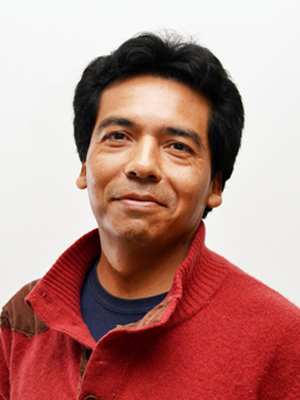# Juan AguilarDivision of Actuarial Science, Statistics and Mathematics
Department of Mathematics

# Juan Aguilar

Full time professor

I studied the Bachelor of Mathematics at the University of Guanajuato; the subject of my thesis was about wavelets and signal processing. I obtained my Ph.D. in Mathematics from the Courant Institute of Mathematical Sciences at New York University (NYU). In my doctoral thesis I developed algorithms and error estimators to approximate the solution of certain partial differential equations with the finite element method. In a postdoctoral stay at the same institute I worked on the numerical approximation of solutions of integral equations that model the dispersion of acoustic waves. I joined the Department of Mathematics at ITAM in the year 2000.